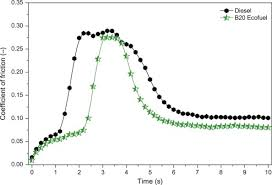## How to Calculate and Solve for Co-efficient allowing for Friction | Design of Gating SystemThe image above represents co-efficient allowing for friction.

To compute for co-efficient allowing for friction, two essential parameters are needed and these parameters are Friction Co-efficient of the Gating System (μ1) and Friction Co-efficient of the Mould (μ2).

The formula for calculating co-efficient allowing for friction:

μ = μ1 x μ2

Where:

μ = Co-efficient allowing for Friction
μ1 = Friction Co-efficient of the Gating System
μ2 = Friction Co-efficient of the Mould

Let’s solve an example;
Find the co-efficient allowing for friction when the friction co-efficient of the gating system is 10 and the friction co-efficient of the mould is 14.

This implies that;

μ1 = Friction Co-efficient of the Gating System = 10
μ2 = Friction Co-efficient of the Mould = 14

μ = μ1 x μ2
μ = 10 x 14
μ = 140

Therefore, the co-efficient allowing for friction is 140.

Calculating the Friction Co-efficient of the Gating System when the Co-efficient Allowing for Friction and the Friction Co-efficient of the Mould is Given.

μ1 = μ / μ2

Where;

μ1 = Friction Co-efficient of the Gating System
μ = Co-efficient allowing for Friction
μ2 = Friction Co-efficient of the Mould

Let’s solve an example;
Find the friction co-efficient of the gating system when the co-efficient allowing for friction is 45 and the friction co-efficient of the mould is 15.

This implies that;

μ = Co-efficient allowing for Friction – 45
μ2 = Friction Co-efficient of the Mould = 15

μ1 = μ / μ2
μ1 = 45 / 15
μ1 = 3

Therefore, the friction co-efficient of the gating system is 3.

Calculating the Friction Co-efficient of the Mould when the Co-efficient Allowing for Friction and the Friction Co-efficient of the Gating System is Given.

μ2 = μ / μ1

Where;

μ2 = Friction Co-efficient of the Mould
μ = Co-efficient allowing for Friction
μ1 = Friction Co-efficient of the Gating System

Let’s solve an example;
Find the friction co-efficient of the mould when the co-efficient allowing for friction is 50 and the friction co-efficient of the gating system is 25.

This implies that;

μ = Co-efficient allowing for Friction = 50
μ1 = Friction Co-efficient of the Gating System = 25

μ2 = μ / μ1
μ2 = 50 / 25
μ2 = 2

Therefore, the friction co-efficient of the mould is 2.